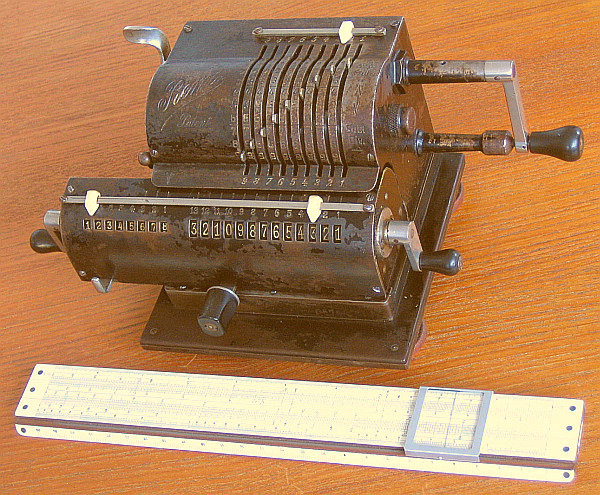previous <<==>> next

REMA
( =  ACRONYM   for   REchenMAschine )
Have a Look Inside ...The contemporary NESTLER No.23 (= 25 cm / 10" ) demonstrates REMA's compactness
 ``` T E C H N I C A L D A T A of the Mechanical Calculator » R E M A « ************************************************************************** Serial Number: 6578 Dimensions: (ca.) Width = 9 " / 23 cm Depth = 5_1/2 " / 14 cm Height = 4_3/4 " / 12 cm Weight: (ca.) 10 lbs / 4.5 kg Mechanics: Pin-Wheel / Sprossenrad 10s Carry Mechanism Counter Stepping Mechanism + Animation Functions: Add, Subtract, Multiply, Divide Registers: Input = 9 Decimals Counter = 8 Decimals (NO Carry; Neg.Figures RED) Arithmetic = 13 Decimals Manufacturer: Braunschweiger Rechenmaschinen Fabrik REMA GmbH Braunschweig / Germany 1923 H O W T O U S E the R E M A *********************************** BASIC SETTINGS: =============== (a) Crank: ---------- For addition (+) the crank is turned clockwise; for subtraction (-) the crank is turned counter-clockwise. In idle position the crank is down & locked. To make one or more turns with the crank, pull out the handle to unlock. When finished the turn(s), let the handle snap into the lock again. The locked down position only makes other functions accessible. REMARK: Every started turn has to be finished completely! Accidentally started turns are correctible somehow. (b) Clearing Registers: ----------------------- The input unit Pull the SILVER LEVER on the left side The counter unit will be cleared with the CRANK on the LEFT SIDE of the carriage The arithmetic register will be cleared with the CRANK on the RIGHT SIDE of the carriage (c) Shifting the Carriage: -------------------------- Pushing the BLACK KNOB on front to the RIGHT, will move the carriage step-by-step to the right. The carriage can be moved continuously to the left, when the LEFT HAND hold the carriage's left side AND the LEFT THUMB press the little SILVER LEVER to the LEFT. The left position "1" is the "Start Position" of the carriage. (d) NON-Counting: ----------------- When the little ball on the left side of the case is pushed down, the counter is disconnected - e.g. for DIVISION Procedure ( s. below ). ADDITION & SUBTRACTION: ======================= Example: 123 + 45 - 6 = 162 Clear input, counter and arithmetic units. ADD: Enter the first number (123) in the far right of the input unit. Make a positive (clockwise) turn with the crank to transfer the number into arithmetic unit. The counting unit displays the figure 1. Enter the second number (45). Make a positive (clockwise) turn with the crank to add the number. The arithmetic unit displays the intermediate sum (168) and the counting unit displays the figure 2. SUBTRACT: Enter the third number (6). Make a negative (counter-clockwise) turn with the crank. The arithmetic unit displays the result (162) and the counting unit is decreased by 1. REMARK: NEGATIVE RESULTS are displayed in the arithmetic unit as the COMPLEMENT of the next higher 10, 100, 1000, ... Example: -12 = 99...9988 MULTIPLICATION: =============== Example: 123 x 45 = 5535 Clear input, counter and arithmetic units. Enter the multiplicand (123) in the far right of the input unit. The multiplicator (45) has two digits, so the carriage is shifted to position 2. Make positive (clockwise) turns with the crank, until the first figure of the multiplicator (4) will appear in the 2nd position of the counter unit. Shift the carriage to position 1. Repeat making positive turns with the crank, until the second figure of the multiplicator (5) appears in the 1st position of the counter unit. The multiplication is done: The multiplicand (123) stays in the input unit, the multiplicator (45) in the counter and the result (5535) is in the arithmetic unit. DIVISION: ========= Example: 22 : 7 = 3.1428571 Remainder 3 Division requires 3 steps: (1st) Set the Dividend into Arithmetic Unit: -------------------------------------------- For the maximum number of decimals, pull out the carriage to the far right position. Enter the dividend (22) in the far right of the input unit. Push the "NON-COUNT-LEVER" and make a positive (clockwise) turn with the crank to transfer the divident into arithmetic unit. (2nd) Set the Divisor into Input Unit: -------------------------------------- Enter the divisor (7) above the dividend (22). (3rd) Divide: ------------- Make negative (counter-clockwise) turns with the crank until the arithmetic unit shows an "underflow". Make one positive (clockwise) turn with the crank. Move the carriage to the next left position. Repeat this procedure until the required number of decimals ... The result (3.1428571) is in the counter unit ( in RED ), and the remainder (3) is in the arithmetic unit. The divisor (7) stays in the input unit, therefore an additional decimal can be estimated ... ( 4, cause 4 x 7 = 28). R E M A R K S : --------------- The COUNTER-FIGURES ( WHITE/RED ) follows the "ODHNER-TYPE"-Scheme Have a look at "Calculating Trickies" ... impressum: ************************************************************************** © C.HAMANN http://public.BHT-Berlin.de/hamann 03/04/15 ```